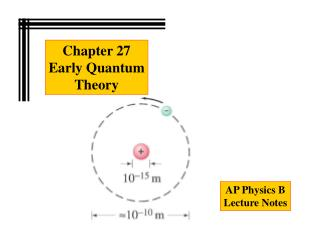# Chapter 27 Early Quantum Theory - PowerPoint PPT PresentationDownload PresentationChapter 27 Early Quantum Theory

Chapter 27 Early Quantum TheoryDownload Presentation## Chapter 27 Early Quantum Theory

- - - - - - - - - - - - - - - - - - - - - - - - - - - E N D - - - - - - - - - - - - - - - - - - - - - - - - - - -
##### Presentation Transcript

1. Chapter 27 Early Quantum Theory AP Physics B Lecture Notes

2. Quantum Physics Sections Blackbody Radiation and Plank’s Hypothesis The Photoelectric Effect and the Particle Theory of Light X-Rays The Compton Effect The The Dual Nature of Light and Matter

3. Blackbody Radiation and Plank’s Hypothesis Stefan-Boltzmann equation Stefan-Boltzmann constant All objects emit radiation whose total intensity is proportional to the fourth power of their temperature. This is called thermal radiation; a blackbody is one that emits thermal radiation only. Power Absolute temperature Surface area Emissivity The spectrum of blackbody radiation has been measured; it is found that the frequency of peak intensity increases linearly with temperature.

4. Blackbody Radiation and Plank’s Hypothesis This figure shows blackbody radiation curves for three different temperatures. Note that frequency increases to the left.

5. Blackbody Radiation and Plank’s Hypothesis The constant h is now called Planck’s constant. This spectrum could not be reproduced using 19th-century physics. A solution was proposed by Max Planck in 1900: The energy of atomic oscillations within atoms cannot have an arbitrary value; it is related to the frequency: Planck’s proposal was that the energy of an oscillation had to be an integral multiple of hf. This is called the quantization of energy.

6. The Photoelectric Effect and the Particle Theory of Light Einstein suggested that, given the success of Planck’s theory, light must be emitted in small energy packets: These tiny packets, or particles, are called photons.

7. The Photoelectric Effect and the Particle Theory of Light Photoelectric equation The photoelectric effect: If light strikes a metal, electrons are emitted. The effect does not occur if the frequency of the light is too low; the kinetic energy of the electrons increases with frequency.

8. The Photoelectric Effect and the Particle Theory of Light If light is a wave, theory predicts: a. Number of electrons and their energy should increase with intensity. b. Frequency would not matter. If light is particles, theory predicts: a. Increasing intensity increases number of electrons but not energy. b. Above a minimum energy required to break atomic bond, kinetic energy will increase linearly with frequency. c. There is a cutoff frequency below which no electrons will be emitted, regardless of intensity.

9. The Photoelectric Effect and the Particle Theory of Light Plotting the kinetic energy vs. frequency: The particle theory assumes that an electron absorbs a single photon. This shows clear agreement with the photon theory, and not with wave theory.

10. X-Rays Electron Charge e High Voltage DV Photon Energy hf

11. The Compton Effect Conservation of Energy Recoiling Electron

12. Chapter 27: Quantum Physics When a photon is scattered from an electron, there will be an increase in its (A) energy. (B) frequency. (C) wavelength. (D) momentum.

13. The Dual Nature of Light and Matter Clearly, a photon must travel at the speed of light. Looking at the relativistic equation for momentum, it is clear that this can only happen if its rest mass is zero. We already know that the energy is hf; we can put this in the relativistic energy-momentum relation and find the momentum:

14. The Dual Nature of Light and Matter Photons passing through matter can undergo the following interactions: A. The photon can produce an electron-positron pair B. Photoelectric effect: photon is completely absorbed, electron is ejected C. Photon may be totally absorbed by electron, but not have enough energy to eject it; the electron moves into an excited state D. The photon can scatter from an atom and lose some energy

15. The Dual Nature of Light and Matter In pair production, energy, electric charge, and momentum must all be conserved. Energy will be conserved through the mass and kinetic energy of the electron and positron; their opposite charges conserve charge; and the interaction must take place in the electromagnetic field of a nucleus, which can contribute momentum.

16. The Dual Nature of Light and Matter What is the minimum photon energy needed to produce a m+ and m- pair? The mass of each (muon) is 207 times the mass of the electron. What is the wavelength of such a photon?

17. The Dual Nature of Light and Matter Just as light sometimes behaves as a particle, matter sometimes behaves like a wave. The wavelength of a particle of matter is: This wavelength is extraordinarily small. The wave nature of matter becomes more important for very light particles such as the electron.

18. The Dual Nature of Light and Matter What is the wavelength of a neutron ( m = 1.67 x 10-27 kg ) traveling at 6.5 x 104 m/s.

19. The Dual Nature of Light and Matter What is the wavelength of an electron of energy 100 eV?

20. END